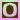Go to content
This Java applet lets you explore various aspects of sampling distributions. When the applet begins, a histogram of a normal distribution is displayed at the topic of the screen. The distribution portrayed at the top of the screen is the population from which samples are taken. The mean of the distribution is indicated by a small blue line and the median is indicated by a small purple line. Since the mean and median are the same, the two lines overlap. The red line extends from the mean one standard deviation in each direction. Note the correspondence between the colors used on the histogram and the statistics displayed to the left of the histogram. The second histogram displays the sample data. This histogram is initially blank. The third and fourth histograms show the distribution of statistics computed from the sample data. The number of samples (replications) that the third and fourth histograms are based on is indicated by the label "Reps=."
 Click:## Glossary

### histogram

A statistical diagram for continuous data similar to a bar chart but where the area of each bar is proportional to the frequency.

### mean

the sum of all the members of the list divided by the number of items in the list.

### median

the middle value of a set of data that has been arranged in order of magnitude; half the data values are above it and half are below.

### normal distribution

A key distribution in statistics which is used to model many naturally occurring phenomena, such as heights and weights; its distribution is bell-shaped.

### population

A collection, group or set of individuals or objects.

### sample

A collection of sampling units drawn from a sampling frame.

### standard deviation

The square root of the variance; a measure of dispersion.

Full Glossary List

## This question appears in the following syllabi:

SyllabusModuleSectionTopicExam Year
AQA A-Level (UK - Pre-2017)S1EstimationCentral Limit Theorem-
AQA A2 Further Maths 2017StatisticsCentral Limit Theorem - ExtraCentral Limit Theorem-
AQA AS/A2 Further Maths 2017StatisticsCentral Limit Theorem - ExtraCentral Limit Theorem-
CCEA A-Level (NI)S2EstimationCentral Limit Theorem-
CIE A-Level (UK)S2EstimationCentral Limit Theorem-
Edexcel A-Level (UK - Pre-2017)S3EstimationCentral Limit Theorem-
Edexcel A2 Further Maths 2017Further Statistics 1Central Limit TheoremCentral Limit Theorem-
Edexcel AS/A2 Further Maths 2017Further Statistics 1Central Limit TheoremCentral Limit Theorem-
I.B. Higher Level7EstimationCentral Limit Theorem-
Methods (UK)M15EstimationCentral Limit Theorem-
OCR A-Level (UK - Pre-2017)S2EstimationCentral Limit Theorem-
OCR A2 Further Maths 2017StatisticsHypothesis Tests and Confidence IntervalsCentral Limit Theorem-
OCR MEI A2 Further Maths 2017Statistics BSample Mean and Central Limit TheoremCentral Limit Theorem-
OCR-MEI A-Level (UK - Pre-2017)S3EstimationCentral Limit Theorem-
Universal (all site questions)EEstimationCentral Limit Theorem-
WJEC A-Level (Wales)S2EstimationCentral Limit Theorem-